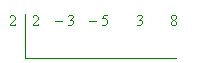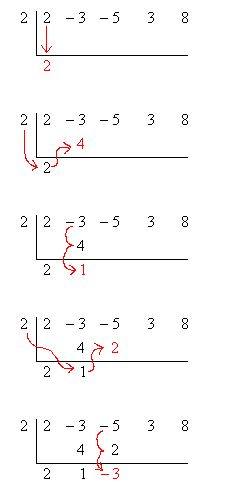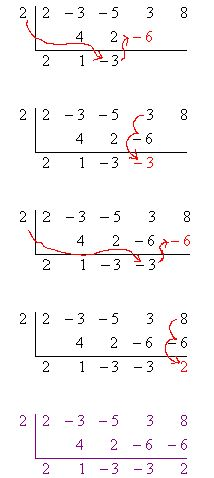Email us to get an instant 20% discount on highly effective K-12 Math & English kwizNET Programs!

#### Online Quiz (WorksheetABCD)

Questions Per Quiz = 2 4 6 8 10

### High School Mathematics - 212.1 Finding Factors Using Synthetic Division

 Synthetic division is a shorthand, or shortcut, method of polynomial division in the special case of dividing by a linear factor (and only works in this case). It is generally used, however, not for dividing out factors but for finding zeroes (or roots) of polynomials. Example:Example: Divide 3x3 – 2x2 + 3x – 4 by x – 3 using synthetic division. Write the answer in the form " q(x) + r(x)/d(x) ". Solution:The remainder is 68 and hence Answer : 3x2 + 7x + 24 + 68/x-3 Directions: Solve the following.
 Q 1: 2x4 – 3x3 – 5x2 + 3x + 8 by x – 2Answer: Q 2: Answer: Q 3: Answer: Q 4: Answer: Q 5: Answer: Q 6: x2 + 5x + 6 by x-1Answer: Q 7: Answer: Q 8: Answer: Question 9: This question is available to subscribers only! Question 10: This question is available to subscribers only!

#### Subscription to kwizNET Learning System offers the following benefits:

• Unrestricted access to grade appropriate lessons, quizzes, & printable worksheets
• Instant scoring of online quizzes
• Progress tracking and award certificates to keep your student motivated
• Unlimited practice with auto-generated 'WIZ MATH' quizzes